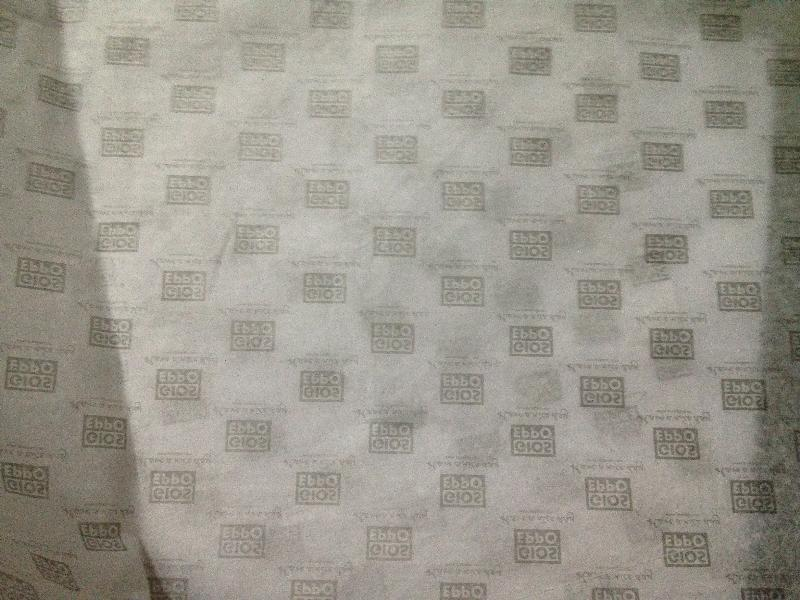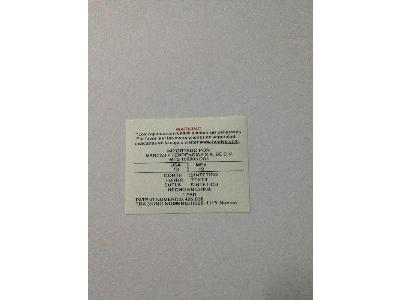• 湖南
• 长沙市
• 常德市
• 郴州市
• 衡阳市
• 怀化市
• 娄底市
• 邵阳市
• 湘潭市
• 湘西土家族苗族自治州
• 益阳市
• 永州市
• 岳阳市
• 张家界市
• 株洲市
• 山西
• 长治市
• 大同市
• 晋城市
• 晋中市
• 临汾市
• 吕梁市
• 朔州市
• 太原市
• 忻州市
• 阳泉市
• 运城市
• 安徽
• 安庆市
• 蚌埠市
• 亳州市
• 巢湖市
• 池州市
• 滁州市
• 阜阳市
• 合肥市
• 淮北市
• 淮南市
• 黄山市
• 六安市
• 马鞍山市
• 宿州市
• 铜陵市
• 芜湖市
• 宣城市
• 广西
• 百色市
• 北海市
• 崇左市
• 防城港市
• 贵港市
• 桂林市
• 河池市
• 贺州市
• 来宾市
• 柳州市
• 南宁市
• 钦州市
• 梧州市
• 玉林市
• 河南
• 安阳市
• 鹤壁市
• 焦作市
• 开封市
• 洛阳市
• 漯河市
• 南阳市
• 平顶山市
• 濮阳市
• 三门峡市
• 商丘市
• 新乡市
• 信阳市
• 许昌市
• 郑州市
• 周口市
• 驻马店市
• 吉林
• 白城市
• 白山市
• 长春市
• 吉林市
• 辽源市
• 四平市
• 松原市
• 通化市
• 延边朝鲜族自治州
• 广东
• 潮州市
• 东莞市
• 佛山市
• 广州市
• 河源市
• 惠州市
• 江门市
• 揭阳市
• 茂名市
• 梅州市
• 清远市
• 汕头市
• 汕尾市
• 韶关市
• 深圳市
• 阳江市
• 云浮市
• 湛江市
• 肇庆市
• 中山市
• 珠海市
• 辽宁
• 鞍山市
• 本溪市
• 朝阳市
• 大连市
• 丹东市
• 抚顺市
• 阜新市
• 葫芦岛市
• 锦州市
• 辽阳市
• 盘锦市
• 沈阳市
• 铁岭市
• 营口市
• 湖北
• 鄂州市
• 恩施土家族苗族自治州
• 黄冈市
• 黄石市
• 荆门市
• 荆州市
• 直辖行政单位
• 十堰市
• 随州市
• 武汉市
• 咸宁市
• 襄阳市
• 孝感市
• 宜昌市
• 江西
• 抚州市
• 赣州市
• 吉安市
• 景德镇市
• 九江市
• 南昌市
• 萍乡市
• 上饶市
• 新余市
• 宜春市
• 鹰潭市
• 浙江
• 杭州市
• 湖州市
• 嘉兴市
• 金华市
• 丽水市
• 宁波市
• 衢州市
• 绍兴市
• 台州市
• 温州市
• 舟山市
• 青海
• 果洛藏族自治州
• 海北藏族自治州
• 海东地区
• 海南藏族自治州
• 海西蒙古族藏族自治州
• 黄南藏族自治州
• 西宁市
• 玉树藏族自治州
• 甘肃
• 白银市
• 定西市
• 甘南藏族自治州
• 嘉峪关市
• 金昌市
• 酒泉市
• 兰州市
• 临夏回族自治州
• 陇南市
• 平凉市
• 庆阳市
• 天水市
• 武威市
• 张掖市
• 贵州
• 安顺市
• 毕节市
• 贵阳市
• 六盘水市
• 黔东南苗族侗族自治州
• 黔南布依族苗族自治州
• 黔西南布依族苗族自治州
• 铜仁地区
• 遵义市
• 陕西
• 安康市
• 宝鸡市
• 汉中市
• 商洛市
• 铜川市
• 渭南市
• 西安市
• 咸阳市
• 延安市
• 榆林市
• 西藏
• 阿里地区
• 昌都地区
• 拉萨市
• 林芝地区
• 那曲地区
• 日喀则地区
• 山南地区
• 宁夏
• 固原市
• 石嘴山市
• 吴忠市
• 银川市
• 中卫市
• 福建
• 福州市
• 龙岩市
• 南平市
• 宁德市
• 莆田市
• 泉州市
• 三明市
• 厦门市
• 漳州市
• 内蒙古
• 阿拉善盟
• 巴彦淖尔市
• 包头市
• 赤峰市
• 鄂尔多斯市
• 呼和浩特市
• 呼伦贝尔市
• 通辽市
• 乌海市
• 乌兰察布市
• 锡林郭勒盟
• 兴安盟
• 云南
• 保山市
• 楚雄彝族自治州
• 大理白族自治州
• 德宏傣族景颇族自治州
• 迪庆藏族自治州
• 红河哈尼族彝族自治州
• 昆明市
• 丽江市
• 临沧市
• 怒江傈僳族自治州
• 曲靖市
• 思茅市
• 文山壮族苗族自治州
• 西双版纳傣族自治州
• 玉溪市
• 昭通市
• 新疆
• 阿克苏地区
• 阿勒泰地区
• 巴音郭楞蒙古自治州
• 博尔塔拉蒙古自治州
• 昌吉回族自治州
• 哈密地区
• 和田地区
• 喀什地区
• 克拉玛依市
• 克孜勒苏柯尔克孜自治州
• 直辖行政单位
• 塔城地区
• 吐鲁番地区
• 乌鲁木齐市
• 伊犁哈萨克自治州
• 黑龙江
• 大庆市
• 大兴安岭地区
• 哈尔滨市
• 鹤岗市
• 黑河市
• 鸡西市
• 佳木斯市
• 牡丹江市
• 七台河市
• 齐齐哈尔市
• 双鸭山市
• 绥化市
• 伊春市
• 香港
• 香港
• 九龙
• 新界
• 澳门
• 澳门
• 其它地区
• 台湾
• 台中市
• 台南市
• 高雄市
• 台北市
• 基隆市
• 嘉义市
•拷贝纸生产厂商-泉州物超所值的拷贝纸供应

品牌:德弘,,

出厂地:鹿寨县(鹿寨镇)

报价：面议

晋江德弘包装用品有限公司

黄金会员：主营：TPU鞋舌标,转印标厂家直销,吊牌定制,包装纸生产商,鞋盒批发

•新闻纸生产厂家-博大纸业供应同行中出色的新闻纸

品牌:博大,晨鸣,华泰

出厂地:凤山县(凤城镇)

报价：面议

青州博大纸业贸易有限公司

黄金会员：主营：铜版纸,轻涂纸,白卡纸,双胶纸,书写纸

•铜版卡纸生产商-潍坊质量好的铜版纸供应

品牌:博大,晨鸣,华泰

出厂地:凤山县(凤城镇)

报价：面议

青州博大纸业贸易有限公司

黄金会员：主营：铜版纸,轻涂纸,白卡纸,双胶纸,书写纸

•金东A4纸|哪里能买到优惠的A4纸

品牌:博大,晨鸣,华泰

出厂地:凤山县(凤城镇)

报价：面议

青州博大纸业贸易有限公司

黄金会员：主营：铜版纸,轻涂纸,白卡纸,双胶纸,书写纸

•转印标厂商|福建实惠的转印标推荐

品牌:德弘,,

出厂地:鹿寨县(鹿寨镇)

报价：面议

晋江德弘包装用品有限公司

黄金会员：主营：TPU鞋舌标,转印标厂家直销,吊牌定制,包装纸生产商,鞋盒批发

•银河新闻纸-质量优的新闻纸生产厂家推荐

品牌:博大,晨鸣,华泰

出厂地:凤山县(凤城镇)

报价：面议

青州博大纸业贸易有限公司

黄金会员：主营：铜版纸,轻涂纸,白卡纸,双胶纸,书写纸

•进口哑粉纸_实惠的哑粉纸，博大纸业提供

品牌:博大,晨鸣,华泰

出厂地:凤山县(凤城镇)

报价：面议

青州博大纸业贸易有限公司

黄金会员：主营：铜版纸,轻涂纸,白卡纸,双胶纸,书写纸

•A4纸生产厂家-潍坊哪里买实用的A4纸

品牌:博大,晨鸣,华泰

出厂地:凤山县(凤城镇)

报价：面议

青州博大纸业贸易有限公司

黄金会员：主营：铜版纸,轻涂纸,白卡纸,双胶纸,书写纸

•省直辖县级行政单位海南折页厂|划算的海南折页，海南万佳达提供

品牌:万佳达,万佳达包装,海南万佳达包装

出厂地:南丹县(城关镇)

报价：面议

海南万佳达包装有限公司

黄金会员：主营：海南纸箱包装,海南精美礼盒,海南手提袋,海南水果箱包装,海南水印箱

•进口双面铜版纸-价格适中的铜版纸产品信息

品牌:博大,晨鸣,华泰

出厂地:凤山县(凤城镇)

报价：面议

青州博大纸业贸易有限公司

黄金会员：主营：铜版纸,轻涂纸,白卡纸,双胶纸,书写纸

• 没有找到合适的供应商？您可以发布采购信息

没有找到满足要求的供应商？您可以搜索 文化印刷用纸批发 文化印刷用纸公司 文化印刷用纸厂

最新入驻厂家

相关产品:
拷贝纸生产厂商 新闻纸生产厂家 铜版卡纸生产商 金东A4纸 转印标厂商 银河新闻纸 进口哑粉纸 A4纸生产厂家 省直辖县级行政单位海南折页厂 进口双面铜版纸